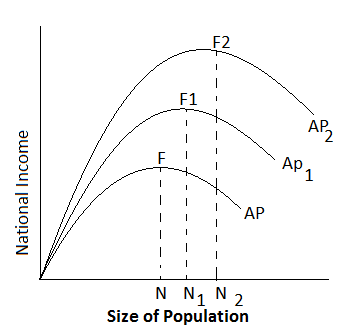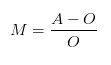# Change in Optimum Population

The optimum population, however, is not fixed, for over a period of time. Conditions are liable to change. If it were possible to increase the supply of other factors with increase in population, the optimum might be raised. Optimum population is relative to resources and technology. So if there is increase in capital stock or natural resources or level of technology. There will be an upward shift in the average and marginal product curves. The per capita output will increase. This means that the level of optimum population too will increase. This has been represented in figure below:As shown in figure, with given resources and technology, the per capita output curve is AP. Here optimum population is and maximum output per capita is NF. When capital and natural resource increase or there is improvement in technology, the per capita output curve shifts upward in the form AP, Now the optimum population is N, and maximum output per capita is N,F,. Here both level of optimum population and maximum output per capita is higher than before. Likewise, further increase in resources and technology will shift the per capita output curve upward in the form of AP2. Now the level of optimum population and maximum per capita output are N2 and N2 F2 respectively which are higher than before.

Dalton has given a formula to measure the extent to which the actual population of a country deviates from the optimum population. The extent of deviation is called maladjustment